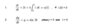# Using the Laplace transform to solve these differential and integral equations from circuits

• Engineering
Homework Statement:
Find i(t) and y(t) in this laplace of circuit formula
Relevant Equations:
Laplace transform to find i(t) and y(t)
im not understand what to do with this formula :

#### Attachments

•assignment.jpg
5.2 KB · Views: 140

Homework Helper
Transform the integro-differential equations to the Laplace domain using known properties.
In the Laplace domain the equations can be solves algebraically.
Transform the solution back to the time domain.
useful properties
$$L\{ \mathrm{f} (t) \}= \mathrm{F}(s)$$
$$L\{ \mathrm{f}^\prime (t) \}=s \, \mathrm{F}(s)-\mathrm{f} (0^+)$$
$$L\left\{ \int_{0^+}^t \mathrm{f} (u) du \right\}=\frac{1}{s} \, \mathrm{F}(s)$$

•••